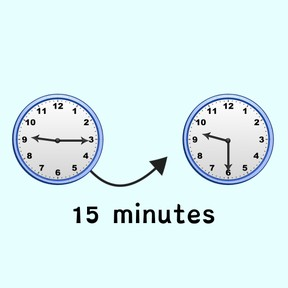Time difference between analog clocks with quarters

# Time difference between analog clocks with quarters

I can determine the time difference between analog clocks with quarter hours.8,000 schools use Gynzy92,000 teachers use Gynzy1,600,000 students use Gynzy

## General

In this lesson, students will practice calculating elapsed time with quarter hours using analog clocks. Students will tell what the difference is between two clocks and recognize how long it will take until it is a given time. Students will watch a video all about elapsed time. Then students will learn how to use number lines and T-charts when solving word problems in a series of elapsed problem activities.

## Standards

CCSS.Math.Content.3.MD.A.1

## Learning Objective

Students will be able to properly tell what the difference is between two clocks with quarter hours and determine how long a time is between them.

## Introduction

Students review analog clocks and their importance. Then students go over quarter hours and practice reading quarter hours on analog clocks before the time runs out.

## Instruction

The lesson will begin with an explanation of elapsed time. Have students watch a video about solving elapsed time word problems with timelines. Guide students in solving an example elapsed time question using both timelines and T-charts. Then, guide students in completing several activities in which they practice solving elapsed time problems such as filling in the chart to show the correct time elapsed between the start and end of races. Have students further test their time-solving skills in an elapsed time Gynzy game show.

## Quiz

Students respond to ten multiple-choice questions. They will read the time on two analog clocks then determine the correct elapsed time.

## Closing

Students will write the name of their favorite activity, and adjust analog clocks to reflect the start and end time of that activity. Finally, students will calculate the time elapsed between the two times.

### The online teaching platform for interactive whiteboards and displays in schools

• Save time building lessons

• Manage the classroom more efficiently

• Increase student engagement